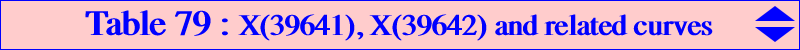The real foci of the Brocard inellipse are the Brocard points Ω1, Ω2. They are a bicentric pair, with barycentrics 1/b^2 : 1/c^2 : 1/a^2 and 1/c^2 : 1/b^2 : 1/a^2, denoted by PU(1) in ETC, see Bicentric Pairs of Points. In contrast to the real foci, the imaginary foci X(39641), X(39642) of the Brocard inellipse are major triangle centers. They both lie on the Brocard axis OK, on the Kiepert circumhyperbola, and on the curves in the table below. They are obviously isogonal conjugates in ABC and symmetric in X(39). They are also inverse in the Brocard circle, and isogonal conjugates in the circumcevian triangle of K. X(39641) = a^2(S + i SA) : b^2(S + i SB) : c^2(S + i SC) and X(39642) = a^2(S - i SA) : b^2(S - i SB) : c^2(S - i SC), with S = 2 area(ABC). In KHO-coordinates, they are {√3, 0, i} and {-√3, 0, i}, see CL075.cubics isogonal Ω1, Ω2 circum type K003 yes pK, stelloid, CircumNormal triangle K019 yes yes nK0, focal K049 K373 pK, stelloid K102 yes pK, Grebe triangle K115 stelloid K166 yes yes nK, focal K248 yes yes nK, focal K268 stelloid K326 yes yes pK K359 yes yes nK, strophoid K369 no nodal, K373 K049 pK, CircumNormal triangle K444 K512 yes psK(X32, X6, X4) K512 K444 yes psK(X2, X2, X3) K516 yes stelloid K587 nodal K588 yes cK(#X1, X101) K643 stelloid, Grebe triangle K688 yes yes nK, focal K708 stelloid K731 spK, Grebe triangle K763 yes pK K801 no nodal, K828 K1283 psK, circular K833 no stelloid, central, K1096 no circular, K1098 no stelloid, K1103 yes no ITB triangle K1113 no K1139 stelloid K1200 no crunodal, K1283 K828 psK, circular other curves Q019 Q094 circular quartic Q039 yes bicircular sextic Q065 equilateral quintic Q073 quartic Q088 quartic Q094 Q019 circular quintic Q138 yes circular sextic Q157 Q158 yes circular quintic Q158 Q157 yes circular quartic Q167 quartic Q168 quartic Q171 yes sexticNotes • the cubics in the yellow cells are those of Table 51. They are the McCay stelloids spK(X3,Q). Their isogonal transforms are CircumNormal cubics, see Table 25. • the cubics in the green cells are focal nKs, loci of foci of inconics with center on a line passing through X(39), the center of the Brocard inellipse. Every cubic passes through Ω1, Ω2. • the cubics in the orange cells are pKs. See further properties below.pKs passing through X(39641) and X(39642) Since X(39641), X(39642) are two isogonal conjugates on the Brocard axis, it is obvious that every isogonal pK with pivot P on the Brocard axis must contain these points. These cubics are in a same pencil with base-points A, B, C, the in/excenters and X(39641), X(39642). Examples : K003 = pK(X6, X3), K102 = pK(X6, X6), K326 = pK(X6, X39), K763 = pK(X6, X1350). Now, for any point M on the Brocard axis, pK(X54 x M, X54) also passes through X(39641), X(39642). The barycentric product X54 x M lies on the trilinear polar of X(14586) = X(54) x X(110), a line (L) passing through {160, 184, 571, 3135, 11135, 11136, 14533, 14573, 39231, 41270}. Example : K373 = pK(14533, X54). More generally, for any Ω ≠ X(6) and P ≠ X(54), there is one and only one pK(Ω, P) that passes through X(39641), X(39642). Example : K049 = pK(X53, X4). *** With Ω = p:q:r and P = u:v:w, the transformations ΩtoP and PtoΩ are reciprocal and quadratic given by : ΩtoP : p:q:r → b^2*c^2*(b^2 - c^2)*(b^2*c^2*p^2 - a^4*q*r) + a^4*p*(4*SA^2 - b^2*c^2)*(c^2*q - b^2*r) : : , PtoΩ : u:v:w → b^2*c^2*(b^2 - c^2)*(a^2*(b^2 + c^2) - (b^2 - c^2)^2)*u^2 + a^4*b^2*c^2*(b^2 - c^2)*v*w - a^2*u*(c^4*(4*SC^2 - a^2*b^2)*v - b^4*(4*SB^2 - a^2*c^2)*w) : : . ΩtoP has three singular points X6 and two imaginary points Y1 and Y2, with barycentric product X(32), that lie on the line (L) above and on the rectangular circum-hyperbola (H5) passing through X(5). Every point on (L) is sent to X(54) and every point on (H5) is sent to a point on the Kiepert hyperbola. Y1 is the intersection of the lines {X39641,X32046}, {X39642,X5} and Y2 is the intersection of the lines {X39642,X32046}, {X39641,X5}. Moreover, Y1 and Y2 are harmonically conjugated with respect to {X184,X571}. The singular points of PtoΩ are X(54), X(39641), X(39642). Every point on the Brocard axis is sent to X(6). Note that, for every point P on the Kiepert hyperbola, Ω = P x X(5) lies on (H5), hence pK(Ω, P) has its isopivot at X(5) and the tangents at A, B, C, P pass through X(5). This is the case of the stelloid K049 and pK(X5, X2). *** Y1 and Y2 lie on every pK(X32, P) where P is a point on the line (L). They also lie on the nodal cubic K1284 = cK(#X6, X1576). Y1 and Y2 are now X(51539), X(51540) in ETC. They are the barycentric squares of X(39642), X(39641) respectively.